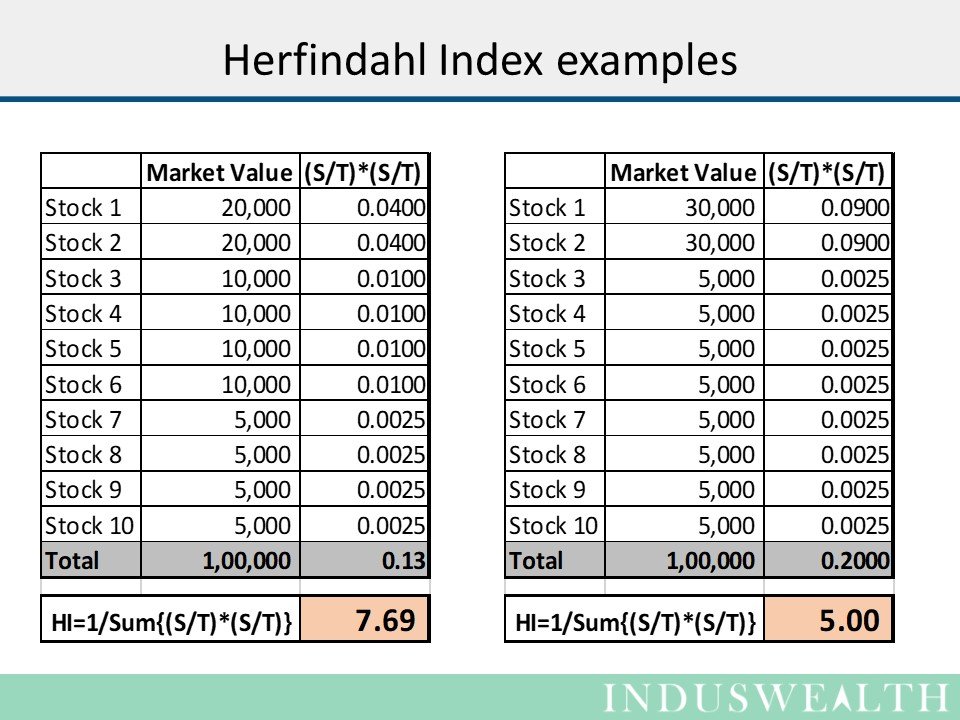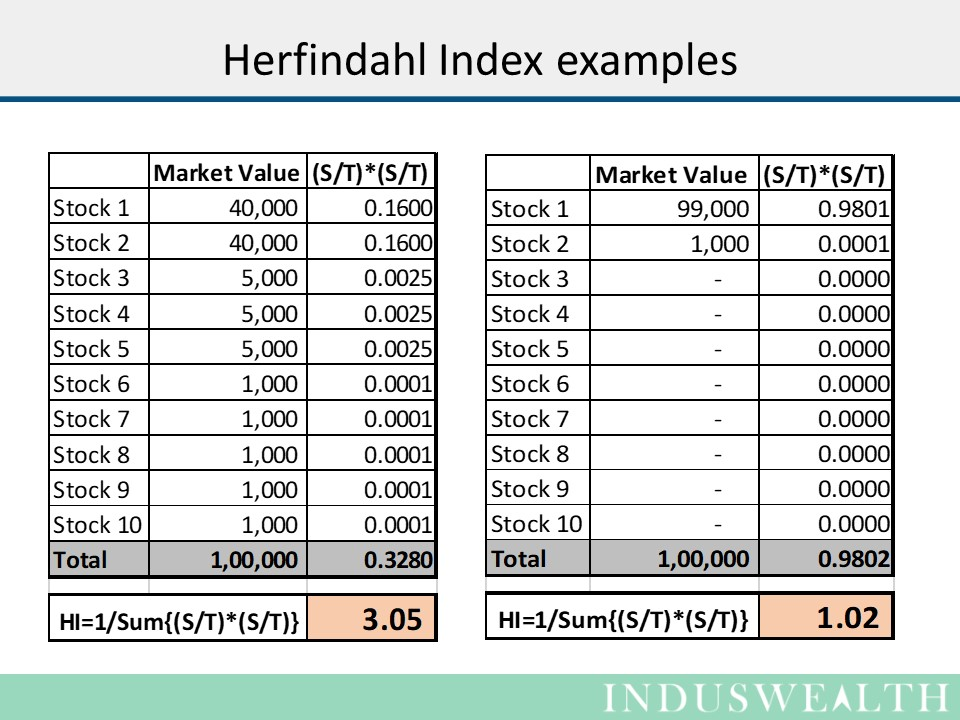# How many stocks do I have in my portfolio?

This seems to a straight forward question, just count the number of stocks and one has the answer. Not so fast!!!

Let’s start with an extreme example, a person has 2 stocks in the portfolio worth 100,000. If the value of stock A is 99,000 and stock B is 1,000.  10% drop in the value of stock A hurts much more than a 10% drop in value of stock B. Does the portfolio really have 2 stocks then?

Its intuitive that it is definitely not 2 stocks, so how do we count the stocks in a portfolio?

There are a few statistical approaches to count the number of stocks. Herfindahl index is one of the better ways.

Let’s take another extreme example and use Herfindahl Index to count the number of stocks. A portfolio of 100,000 composed of 10 stocks each of value 10,000.Herfindahl index also states that there are 10 stocks in the portfolio. All this math to say that there are 10 stocks in the portfolio!!!

Well this just proves that Hefindahl index can do simple counting in complex way.

Let’s look at a few more examples to see if it can do some complex counting in a simple way….The answer to the question we started with, i.e the number of stocks in a 100,000 portfolio with 2 stocks of market value 99,000 and 1,000 is 1.02.

Counting the number of stocks in a portfolio is important to be able to manage it well. We hope you find Herfindahl index a useful tool.

Happy Investing….

## IndusWealth: Making your money work for you